Start typing, then use the up and down arrows to select an option from the list.
2:22 minutes
Problem 5a
Textbook Question

# In a population of 2500, how many babies would you expect to have cystic fibrosis, a homozygous recessive condition, if the frequency of the dominant allele is 0.9 and the population is at Hardy–Weinberg equilibrium? a. 0.9×2500=2250 b. 2×0.9×0.1×2500=450 c. 0.9×0.1×2500=225 d. 0.1x0.1x2500=25Verified Solution
This video solution was recommended by our tutors as helpful for the problem above.
224views

### Watch next

Master Nucleic Acids with a bite sized video explanation from Jason Amores Sumpter

Start learning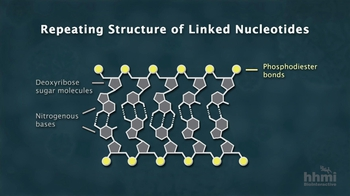11278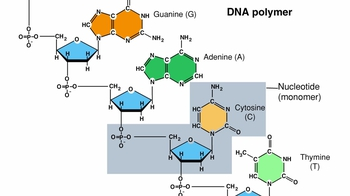91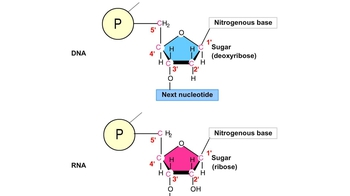19453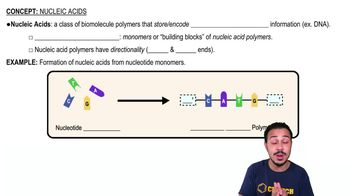03:22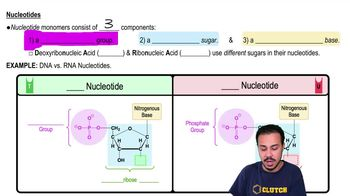05:35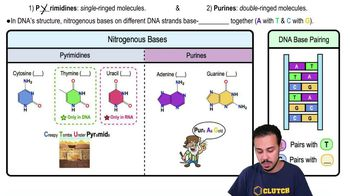07:21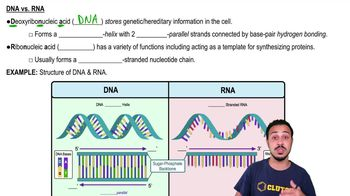05:57